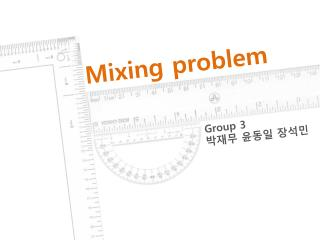DownloadDownload PresentationMixing problem

# Mixing problem

Download Presentation## Mixing problem

- - - - - - - - - - - - - - - - - - - - - - - - - - - E N D - - - - - - - - - - - - - - - - - - - - - - - - - - -
##### Presentation Transcript

1. Mixing problem Group 3 박재무 윤동일 장석민

2. Contents - Briefing conditions - Problem 1-1)Determine the function - Problem 1-2) Approximation of the concentration - Problem 1-3) Compare with example 1 and problem 1

3. Briefing conditions

4. ※ The brine solution in the tank is kept well stirred, let’s assume the concentration of salt in the tank is uniform Briefing conditions Volume – t = 0, 1000 L – Inputting = (6 L/min) – Outputting = (5 L/min) 5 L/min

5. Briefing conditions Substance – t = 0, x = 0 kg –Inputting = (6 L/min)(1 kg/L) = 6 kg/min –Outputting = (5 L/min)( kg/L) = kg/min 5 L/min

6. Problem 1-1)

7. Problem 1-1) Determine the concentration of salt in the tank as function of time Concentration = substance/volume

8. Briefing conditions Volume – t = 0, 1000 L – Inputting = (6 L/min) – Outputting = (5 L/min) 5 L/min

9. Briefing conditions Substance – t = 0, x = 0 kg –Inputting = (6 L/min)(1 kg/L) = 6 kg/min –Outputting = (5 L/min)( kg/L) = kg/min x(t) = substance In tank at time t Concentration = substance/volume 5 L/min

10. Problem 1-1) Determine the concentration of salt in the tank as function of time Using integrating factor First linear differential equation form

11. Problem 1-1) Determine the concentration of salt in the tank as function of time

12. Problem 1-1) Determine the concentration of salt in the tank as function of time

13. Problem 1-1) Determine the concentration of salt in the tank as function of time Concentration = substance/volume Concentration =

14. Q&A

15. Problem 1-2)

16. Problem 1-2) Use the improved Euler’s method and fourth order Runge-Kutta method to approximate the concentration of salt In the tank after 300 minutes Improved Euler’s Method

17. Problem 1-2) Use the improved Euler’s method and fourth order Runge-Kutta method to approximate the concentration of salt In the tank after 300 minutes RungeKutta order 4

18. Problem 1-2) Use the improved Euler’s method and fourth order Runge-Kutta method to approximate the concentration of salt In the tank after 300 minutes ODE 45

19. Problem 1-2) Use the improved Euler’s method and fourth order Runge-Kutta method to approximate the concentration of salt In the tank after 300 minutes

20. Q&A

21. Problem 1-3)

22. Problem 1-3) Compare to example 1, what can you tell about the concentration of salt in the tank when t →∞ 5L/min

23. Problem 1-3) Compare to example 1, what can you tell about the concentration of salt in the tank when t →∞ exampl 1 problem 1 Volume – t = 0, 1000 L t = 0, 1000 L – Inputting = (6 L/min) Inputting = (6 L/min) – Outputting = (6 L/min) Outputting = (5 L/min) v(t) = 1000 v(t) = 1000 + t Substance – t = 0, 1000 L t = 0, 1000 L – Inputting = (6 L/min)(1 kg/L) = 6 kg/min – Outputting = (6 L/min) Outputting = (5 L/min)( kg/L) = kg/min

24. Problem 1-3) Compare to example 1, what can you tell about the concentration of salt in the tank when t →∞ exampl 1 problem 1 concentration – Analytic, Both converge to 1, when t →∞

25. Problem 1-3) Compare to example 1, what can you tell about the concentration of salt in the tank when t →∞ Numerically, Both also converge to 1, when t →∞

26. Q&A

27. Thank You!